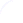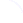Search
 Submission Procedure share: |available in:   PDF (174 kB) PS (164 kB)

get:
 Similar Docs BibTeX Write a comment
get:

DOI:   10.3217/jucs-016-14-1912

### A Heuristic Approach to Positive Root Isolation for Multiple Power Sums

Ming Xu (Chinese Academy of Sciences, China)

Chuandong Mu (East China Normal University, China)

Zhenbing Zeng (East China Normal University, China)

Zhi-bin Li (East China Normal University, China)

Abstract: Given a multiple power sum (extending polynomial's exponents to real numbers), the positive root isolation problem is to find a list of disjoint intervals, satisfying that they contain all positive roots and each of them contains exactly distinct one. In this paper, we develop the pseudo-derivative sequences for multiple power sums, then generalize Fourier's theorem and Descartes' sign rule for them to overestimate the number of their positive roots. Furthermore we bring up some formulas of linear and quadratic complexity to compute complex root bounds and positive root bounds based on Descartes' sign rule and Cauchy's theorem. Besides, we advance a factorization method for multiple power sums with rational coefficients utilizing Q-linear independence, thus reduce the computational complexity in the isolation process. Finally we present an efficient algorithm to isolate all positive roots under any given minimum root separation.

Keywords: Descartes' sign rule, Fourier's theorem, multiple power sums, root bounds, root isolation

Categories: F.2.1, G.1.5